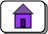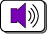created: 07/12/01; audio: 12/05/03; small changes: 06/18/03; 07/20/2015; 09/27/21CHAPTER 14 — Integer Multiplication, Division and Arithmetic Shift Instructions

This chapter discusses the MIPS instructions for performing 32-bit integer multiplication. Some topics of integer representation with bit patterns are reviewed.

Chapter Topics:

• Integer multiplication and division
• The hi and lo registers
• The mult and multu instructions
• The div and divu instructions
• The mfhi and mflo instructions
• Arithmetic shift right
• The sra Instruction

QUESTION 1:

Multiply 9910 times 9910 : .

How many decimal places does each operand (99) have: .

How many decimal places does the result have: .